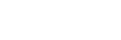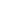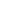# dataset-distillation | Dataset Distillation | Machine Learning library

by   SsnL Python Version: Current License: MIT

by   SsnL Python Version: Current License: MIT

## kandi X-RAY | dataset-distillation Summary

dataset-distillation is a Python library typically used in Artificial Intelligence, Machine Learning, Deep Learning, Pytorch, Tensorflow applications. dataset-distillation has no bugs, it has no vulnerabilities, it has build file available, it has a Permissive License and it has low support. You can download it from GitHub.
Project Page | Paper. We provide a PyTorch implementation of Dataset Distillation. We distill the knowledge of tens of thousands of images into a few synthetic training images called distilled images. (a): On MNIST, 10 distilled images can train a standard LeNet with a fixed initialization to 94% test accuracy (compared to 99% when fully trained). On CIFAR10, 100 distilled images can train a deep network with fixed initialization to 54% test accuracy (compared to 80% when fully trained). (b): We can distill the domain difference between two SVHN and MNIST into 100 distilled images. These images can be used to quickly fine-tune networks trained for SVHN to achieve a high accuracy on MNIST. (c): Our method can be used to create adversarial attack images. If well-optimized networks retrained with these images for one single gradient step, they will catastrophically misclassify a particular targeted class. Dataset Distillation Tongzhou Wang, Jun-Yan Zhu, Antonio Torralba, Alexei A. Efros. arXiv, 2018. Facebook AI Research, MIT CSAIL, UC Berkeley. The code is written by Tongzhou Wang and Jun-Yan Zhu.
Support
Support
Quality
Quality
Security
Security
Reuse
ReuseSupport

• dataset-distillation has a low active ecosystem.
• It has 480 star(s) with 74 fork(s). There are 19 watchers for this library.
• It had no major release in the last 12 months.
• There are 3 open issues and 30 have been closed. On average issues are closed in 16 days. There are no pull requests.
• It has a neutral sentiment in the developer community.
dataset-distillation Support
Best in #Machine Learning
Average in #Machine Learning
dataset-distillation Support
Best in #Machine Learning
Average in #Machine LearningQuality

• dataset-distillation has 0 bugs and 0 code smells.
dataset-distillation Quality
Best in #Machine Learning
Average in #Machine Learning
dataset-distillation Quality
Best in #Machine Learning
Average in #Machine LearningSecurity

• dataset-distillation has no vulnerabilities reported, and its dependent libraries have no vulnerabilities reported.
• dataset-distillation code analysis shows 0 unresolved vulnerabilities.
• There are 0 security hotspots that need review.
dataset-distillation Security
Best in #Machine Learning
Average in #Machine Learning
dataset-distillation Security
Best in #Machine Learning
Average in #Machine LearningLicense

• Permissive licenses have the least restrictions, and you can use them in most projects.
Best in #Machine Learning
Average in #Machine Learning
Best in #Machine Learning
Average in #Machine LearningReuse

• dataset-distillation releases are not available. You will need to build from source code and install.
• Build file is available. You can build the component from source.
• Installation instructions, examples and code snippets are available.
• It has 2666 lines of code, 169 functions and 19 files.
• It has high code complexity. Code complexity directly impacts maintainability of the code.
dataset-distillation Reuse
Best in #Machine Learning
Average in #Machine Learning
dataset-distillation Reuse
Best in #Machine Learning
Average in #Machine Learning
Top functions reviewed by kandi - BETA

kandi has reviewed dataset-distillation and discovered the below as its top functions. This is intended to give you an instant insight into dataset-distillation implemented functionality, and help decide if they suit your requirements.

• Main function .
• Set the state of the experiment .
• Get MNIST dataset .
• Evaluate steps .
• Run k - means training .
• Evaluate the given models .
• Generate visual results .
• Backward computation .
• Wrapper for _call .

## dataset-distillation Key Features

Dataset Distillation

Getting StartedCopyDownload
``````python main.py --mode distill_basic --dataset MNIST --arch LeNet
``````

CitationCopyDownload
``````@article{wang2018dataset,
title={Dataset Distillation},
author={Wang, Tongzhou and Zhu, Jun-Yan and Torralba, Antonio and Efros, Alexei A},
journal={arXiv preprint arXiv:1811.10959},
year={2018}
}
``````

## Community Discussions

Trending Discussions on Machine Learning
###### Trending Discussions on Machine Learning

QUESTION

Using RNN Trained Model without pytorch installed

I have trained an RNN model with pytorch. I need to use the model for prediction in an environment where I'm unable to install pytorch because of some strange dependency issue with glibc. However, I can install numpy and scipy and other libraries. So, I want to use the trained model, with the network definition, without pytorch.

I have the weights of the model as I save the model with its state dict and weights in the standard way, but I can also save it using just json/pickle files or similar.

I also have the network definition, which depends on pytorch in a number of ways. This is my RNN network definition.

``````import torch
import torch.nn as nn
import torch.nn.functional as F
import torch.optim as optim
import random

torch.manual_seed(1)
random.seed(1)
device = torch.device('cpu')

class RNN(nn.Module):
def __init__(self, input_size, hidden_size, output_size,num_layers, matching_in_out=False, batch_size=1):
super(RNN, self).__init__()
self.input_size = input_size
self.hidden_size = hidden_size
self.output_size = output_size
self.num_layers = num_layers
self.batch_size = batch_size
self.matching_in_out = matching_in_out #length of input vector matches the length of output vector
self.lstm = nn.LSTM(input_size, hidden_size,num_layers)
self.hidden2out = nn.Linear(hidden_size, output_size)
self.hidden = self.init_hidden()
def forward(self, feature_list):
feature_list=torch.tensor(feature_list)

if self.matching_in_out:
lstm_out, _ = self.lstm( feature_list.view(len( feature_list), 1, -1))
output_space = self.hidden2out(lstm_out.view(len( feature_list), -1))
output_scores = torch.sigmoid(output_space) #we'll need to check if we need this sigmoid
return output_scores #output_scores
else:
for i in range(len(feature_list)):
cur_ft_tensor=feature_list[i]#.view([1,1,self.input_size])
cur_ft_tensor=cur_ft_tensor.view([1,1,self.input_size])
lstm_out, self.hidden = self.lstm(cur_ft_tensor, self.hidden)
outs=self.hidden2out(lstm_out)
return outs
def init_hidden(self):
return (torch.rand(self.num_layers, self.batch_size, self.hidden_size).to(device),
torch.rand(self.num_layers, self.batch_size, self.hidden_size).to(device))
``````

I am aware of this question, but I'm willing to go as low level as possible. I can work with numpy array instead of tensors, and reshape instead of view, and I don't need a device setting.

Based on the class definition above, what I can see here is that I only need the following components from torch to get an output from the forward function:

• nn.LSTM
• nn.Linear
• torch.sigmoid

I think I can easily implement the sigmoid function using numpy. However, can I have some implementation for the nn.LSTM and nn.Linear using something not involving pytorch? Also, how will I use the weights from the state dict into the new class?

So, the question is, how can I "translate" this RNN definition into a class that doesn't need pytorch, and how to use the state dict weights for it? Alternatively, is there a "light" version of pytorch, that I can use just to run the model and yield a result?

## EDIT

I think it might be useful to include the numpy/scipy equivalent for both nn.LSTM and nn.linear. It would help us compare the numpy output to torch output for the same code, and give us some modular code/functions to use. Specifically, a numpy equivalent for the following would be great:

``````rnn = nn.LSTM(10, 20, 2)
input = torch.randn(5, 3, 10)
h0 = torch.randn(2, 3, 20)
c0 = torch.randn(2, 3, 20)
output, (hn, cn) = rnn(input, (h0, c0))
``````

and also for linear:

``````m = nn.Linear(20, 30)
input = torch.randn(128, 20)
output = m(input)
``````

You should try to export the model using torch.onnx. The page gives you an example that you can start with.

An alternative is to use TorchScript, but that requires torch libraries.

Both of these can be run without python. You can load torchscript in a C++ application https://pytorch.org/tutorials/advanced/cpp_export.html

ONNX is much more portable and you can use in languages such as C#, Java, or Javascript https://onnxruntime.ai/ (even on the browser)

### A running example

Just modifying a little your example to go over the errors I found

Notice that via tracing any if/elif/else, for, while will be unrolled

``````import torch
import torch.nn as nn
import torch.nn.functional as F
import torch.optim as optim
import random

torch.manual_seed(1)
random.seed(1)
device = torch.device('cpu')

class RNN(nn.Module):
def __init__(self, input_size, hidden_size, output_size,num_layers, matching_in_out=False, batch_size=1):
super(RNN, self).__init__()
self.input_size = input_size
self.hidden_size = hidden_size
self.output_size = output_size
self.num_layers = num_layers
self.batch_size = batch_size
self.matching_in_out = matching_in_out #length of input vector matches the length of output vector
self.lstm = nn.LSTM(input_size, hidden_size,num_layers)
self.hidden2out = nn.Linear(hidden_size, output_size)
def forward(self, x, h0, c0):
lstm_out, (hidden_a, hidden_b) = self.lstm(x, (h0, c0))
outs=self.hidden2out(lstm_out)
return outs, (hidden_a, hidden_b)
def init_hidden(self):
return (torch.rand(self.num_layers, self.batch_size, self.hidden_size).to(device).detach(),
torch.rand(self.num_layers, self.batch_size, self.hidden_size).to(device).detach())

# convert the arguments passed during onnx.export call
class MWrapper(nn.Module):
def __init__(self, model):
super(MWrapper, self).__init__()
self.model = model;
def forward(self, kwargs):
return self.model(**kwargs)
``````

Run an example

``````rnn = RNN(10, 10, 10, 3)
X = torch.randn(3,1,10)
h0,c0  = rnn.init_hidden()
print(rnn(X, h0, c0))
``````

Use the same input to trace the model and export an onnx file

``````
torch.onnx.export(MWrapper(rnn), {'x':X,'h0':h0,'c0':c0}, 'rnn.onnx',
dynamic_axes={'x':{1:'N'},
'c0':{1: 'N'},
'h0':{1: 'N'}
},
input_names=['x', 'h0', 'c0'],
output_names=['y', 'hn', 'cn']
)
``````

Notice that you can use symbolic values for the dimensions of some axes of some inputs. Unspecified dimensions will be fixed with the values from the traced inputs. By default LSTM uses dimension 1 as batch.

Next we load the ONNX model and pass the same inputs

``````import onnxruntime
ort_model = onnxruntime.InferenceSession('rnn.onnx')
print(ort_model.run(['y'], {'x':X.numpy(), 'c0':c0.numpy(), 'h0':h0.numpy()}))
``````

Community Discussions, Code Snippets contain sources that include Stack Exchange Network

## Vulnerabilities

No vulnerabilities reported

## Install dataset-distillation

We aim to encapsulate the knowledge of the entire training dataset, which typically contains thousands to millions of images, into a small number of synthetic training images. To achieve this, we optimize these distilled images such that newly initialized network(s) can achieve high performance on a task, after only applying gradient steps on these distilled images. The distilled images can be optimized either for a fixed initialization or random unknown ones from a distribution of initializations. The default options are designed for random initializations. In each training iteration, new initial weights are sampled and trained. Such trained distilled images can be generally applied to unseen initial weights, provided that the weights come from the same initialization distribution. Alternatively, the distilled images can be optimized for a particular initialization, allowing for high performance using even fewer images (e.g., 10 images trains an initialized LeNet to 94% test accuracy).
MNIST: python main.py --mode distill_basic --dataset MNIST --arch LeNet
Cifar10: python main.py --mode distill_basic --dataset Cifar10 --arch AlexCifarNet \ --distill_lr 0.001 AlexCifarNet is an architecture adapted from the cuda-convnet project by Alex Krizhevsky.
MNIST: python main.py --mode distill_basic --dataset MNIST --arch LeNet \ --distill_steps 1 --train_nets_type known_init --n_nets 1 \ --test_nets_type same_as_train
Cifar10: python main.py --mode distill_basic --dataset Cifar10 --arch AlexCifarNet \ --distill_lr 0.001 --train_nets_type known_init --n_nets 1 \ --test_nets_type same_as_train

## Support

For any new features, suggestions and bugs create an issue on GitHub. If you have any questions check and ask questions on community page Stack Overflow .

Explore Related Topics###### Find, review, and download reusable Libraries, Code Snippets, Cloud APIs from over 430 million Knowledge Items
Find more libraries
###### Reuse Solution Kits and Libraries Curated by Popular Use Cases

Save this library and start creating your kit

• © 2022 Open Weaver Inc.
• © 2022 Open Weaver Inc.